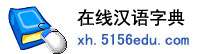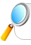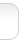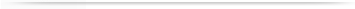汉字   按部首检索  按拼音检索张沛 李沛 刘沛 王沛 陈沛 杨沛 黄沛 赵沛 徐沛 郭沛 宋沛 胡沛 于沛 曾沛 周沛 肖沛 许沛 朱沛 孙沛 田沛 林沛 高沛 程沛 董沛 沈沛 汤沛 曹沛 唐沛 侯沛 马沛

 沛字的男姓名 | 沛字的女姓名 | 沛开头的姓名 | 沛结尾的姓名 | 更多带沛的姓名
 沛 (男) 沛 (女) 沛然 (男) 沛沛 (女) 沛霖 (男) 沛文 (男) 沛林 (男) 沛东 (男) 沛沛 (男) 沛泉 (男) 永沛 (男) 沛华 (男) 沛鑫 (男) 沛峰 (男) 沛源 (男) 沛宇 (男) 沛玲 (女) 沛明 (男) 沛涛 (男) 沛杰 (男) 沛君 (男) 沛军 (男) 沛荣 (男) 文沛 (男) 晓沛 (女) 晓沛 (男) 沛霖 (女) 沛珊 (女) 沛龙 (男) 沛勇 (男) 沛霞 (女) 沛锋 (男) 沛瑶 (女) 沛民 (男) 沛祺 (男) 小沛 (男) 沛权 (男) 明沛 (男) 沛良 (男) 沛强 (男) 沛鸿 (男) 小沛 (女) 成沛 (男) 沛云 (男) 沛辉 (男) 沛洪 (男) 建沛 (男) 沛然 (女) 沛福 (男) 沛新 (男) 沛奇 (男) 沛明 (女) 嘉沛 (男) 沛丰 (男) 润沛 (男) 沛森 (男) 沛松 (男) 兆沛 (男) 沛国 (男) 云沛 (男) 沛达 (男) 沛恒 (男) 沛豪 (男) 沛清 (男) 沛轩 (男) 学沛 (男) 兴沛 (男) 沛刚 (男) 沛伦 (男) 沛文 (女) 文沛 (女) 少沛 (男) 沛亮 (男) 国沛 (男) 仁沛 (男) 沛珍 (女) 沛芬 (女) 沛青 (男) 志沛 (男) 泽沛 (男) 沛缇 (女) 沛芸 (女) 沛莹 (女) 洪沛 (男) 沛和 (男) 沛航 (男) 沛全 (男) 沛红 (女) 丰沛 (男) 沛田 (男) 沛泽 (男) 沛芳 (女) 沛健 (男) 沛春 (男) 沛兴 (男) 沛贤 (男) 清沛 (男) 沛荣 (女) 书沛 (男) 沛云 (女) 楚沛 (男) 培沛 (男) 沛力 (男) 沛宏 (女) 沛华 (女) 沛晴 (女) 沛桓 (男) 沛勋 (男) 沛忠 (男) 沛杨 (男) 沛池 (男) 沛光 (男) 庭沛 (男) 世沛 (男) 沛升 (男) 家沛 (男) 增沛 (男) 沛毅 (男) 沛晓 (男) 沛宁 (男)

 版权所有　在线汉语字典   浙ICP备05019169号